Question

# A 4.0 cm tall object is placed 50.0 cm from a diverging lens having a focal...

A 4.0 cm tall object is placed 50.0 cm from a diverging lens having a focal length of magnitude 20.0cm. a. What is the location of the image? b. What is the magnification of the image? c. Is the image inverted or upright? d. Is the image virtual or real?

Sol:

1/d0+1/di= 1/f
=> 1/du= 1/f - 1/d0
= -1/20cm -1/50cm
=-0.005-0.02=-0.07 cm
=> di = -14.285cm
Minus sign means image is on same side as object, and therefore it must be virtual.
m = -di/d0
= -(-14.285)/50
= 0.2857
So, +0.2857,m=h0/hi
=> h0= m*hi
= 0.2857*4cm
= 1.143 cm
+ means erect, so image is upright and 1.143cm tall.
a) location: same side as object
b) magnification : +0.2857
c) image is UPRIGHT
d) image is VIRTUAL

Hope will get VOTED
thank you

#### Earn Coins

Coins can be redeemed for fabulous gifts.

Similar Homework Help Questions
• ### A 4.0-cm tall object is placed 50.0 cm from a diverging lens having a focal length...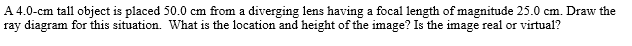A 4.0-cm tall object is placed 50.0 cm from a diverging lens having a focal length of magnitude 25.0 cm. Draw the ray diagram for this situation. What is the location and height of the image? Is the image real or virtual? • Draw an optical axis with the lens centered on the axis. Represent the object at the correct distance from the lens • Draw the three "special rays" from the top of the object • Extend the rays...

• ### Now, a diverging lens with focal length having a magnitude of 20 cm is placed 10...

Now, a diverging lens with focal length having a magnitude of 20 cm is placed 10 cm to the right of the converging lens in problem that has a 2 cm tall object placed 12 cm to the left of a converging lens with focal length of magnitude 15 cm. Determine the location of the final image formed by both lenses (in relation to the diverging lens) and the magnification of the final image. State whether the final image is...

• ### 1.) An object is placed in front of a diverging lens with a focal length of...

1.) An object is placed in front of a diverging lens with a focal length of 17.7 cm. For each object distance, find the image distance and the magnification. Describe each image. (a) 35.4 cm location _____cm magnification _____ nature real virtual upright inverted (b) 17.7 cm location _____  cm magnification _____ nature real virtual upright inverted (c) 8.85 cm location _____ cm magnification _____ nature real virtual upright inverted 2.) An object is placed in front of a converging lens...

• ### A 4.0 cm tall object is placed 50.0 cm from a diverging lens of focal length...

A 4.0 cm tall object is placed 50.0 cm from a diverging lens of focal length 25.0 cm. What is the nature and location of the image? Please include a diagram and work to show the answer. The answers are given as virtual image, 1.3 cm tall, 16.7 cm on the same side as the object but I do not know how to find them.

• ### Ray Optics 3 Problem Statement A 4.0-cm tall object is placed 50.0 cm from a diverging...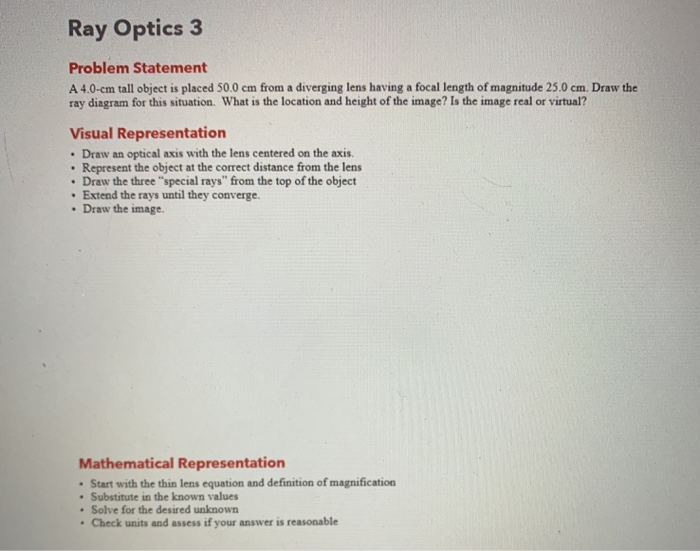Ray Optics 3 Problem Statement A 4.0-cm tall object is placed 50.0 cm from a diverging lens having a focal length of magnitude 25.0 cm. Draw the ray diagram for this situation. What is the location and height of the image? Is the image real or virtual? Visual Representation • Draw an optical axis with the lens centered on the axis. Represent the object at the correct distance from the lens • Draw the three "special rays" from the top...

• ### Ray Optics 3 Problem Statement A 4.0-cm tall object is placed 50.0 cm from a diverging...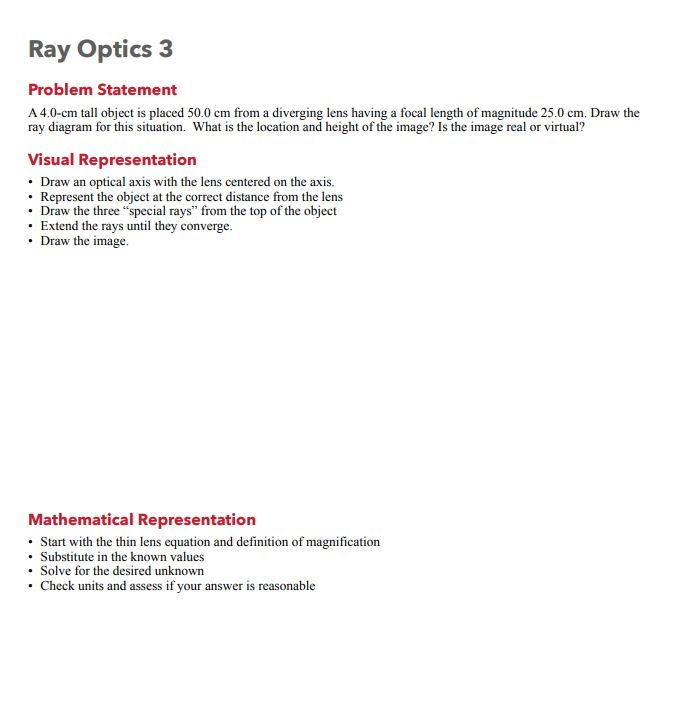Ray Optics 3 Problem Statement A 4.0-cm tall object is placed 50.0 cm from a diverging lens having a focal length of magnitude 25.0 cm. Draw the ray diagram for this situation. What is the location and height of the image? Is the image real or virtual? Visual Representation • Draw an optical axis with the lens centered on the axis. Represent the object at the correct distance from the lens • Draw the three "special rays” from the top...

• ### A real object is 13.6 cm to the left of a thin, diverging lens having a focal length of magnitude 24.5 cm.

A real object is 13.6 cm to the left of a thin, diverging lens having a focal length of magnitude 24.5 cm. (a) is the sign of the focal length negative or positive? negative positive (b) Find the image distance. (c) Find the magnification. (d) State whether the image is real or virtual. real virtual (e) State whether the image is upright or inverted. upright inverted

• ### An object 2.02 cm high is placed 40.2 cm to the left of a converging lens having a focal length o...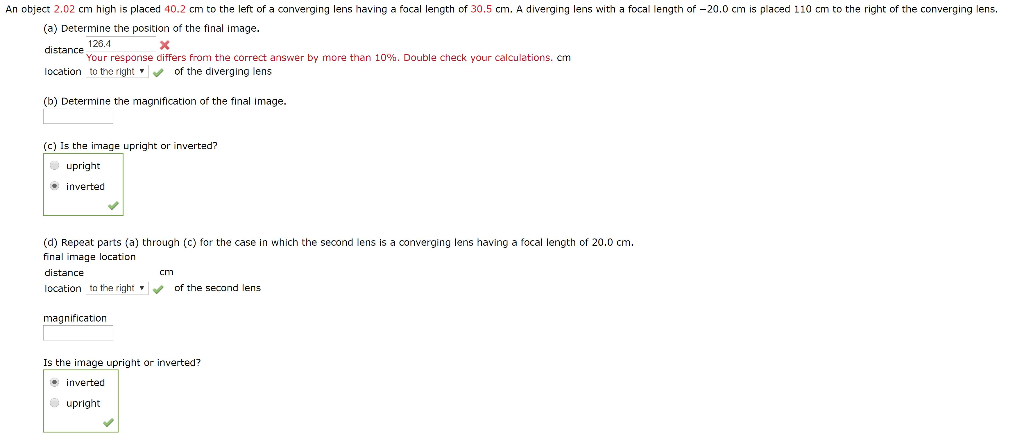An object 2.02 cm high is placed 40.2 cm to the left of a converging lens having a focal length of 30.5 cm. A diverging lens with a focal length of-20.0 cm is placed 110 cm to the right of the converging lens. (a) Determine the position of the final image. distance location to the right , of the diverging lens (b) Determine the magnification of the final image 128.4 Your response differs from the correct answer by more than...

• ### A 4.0 cm tall object is 5.0 cm in front of a diverging lens with a focal length of -6.0 cm. A converging lens with a fo...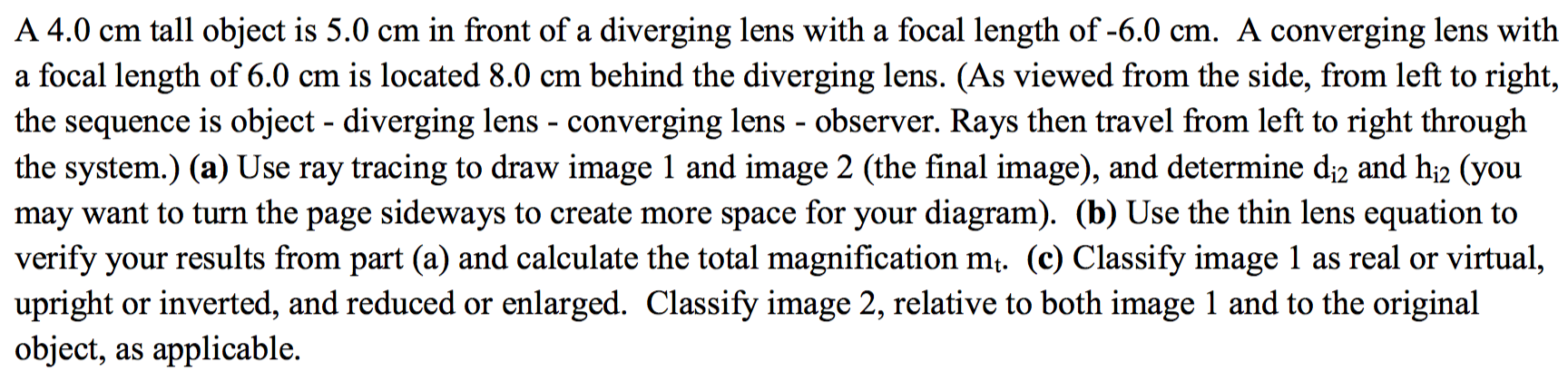A 4.0 cm tall object is 5.0 cm in front of a diverging lens with a focal length of -6.0 cm. A converging lens with a focal length of 6.0 cm is located 8.0 cm behind the diverging lens. (As viewed from the side, from left to right, the sequence is object - diverging lens - converging lens - observer. Rays then travel from left to right through the system.) (a) Use ray tracing to draw image 1 and image...

• ### A 20 cm tall object is located 70 cm away from a diverging lens that has...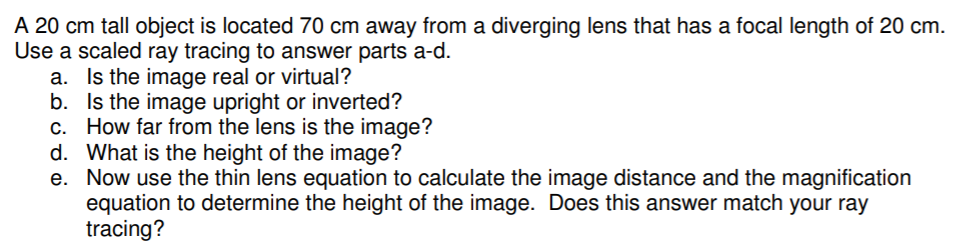A 20 cm tall object is located 70 cm away from a diverging lens that has a focal length of 20 cm. Use a scaled ray tracing to answer parts a-d. a. Is the image real or virtual? b. Is the image upright or inverted? c. How far from the lens is the image? d. What is the height of the image? e. Now use the thin lens equation to calculate the image distance and the magnification equation to determine...